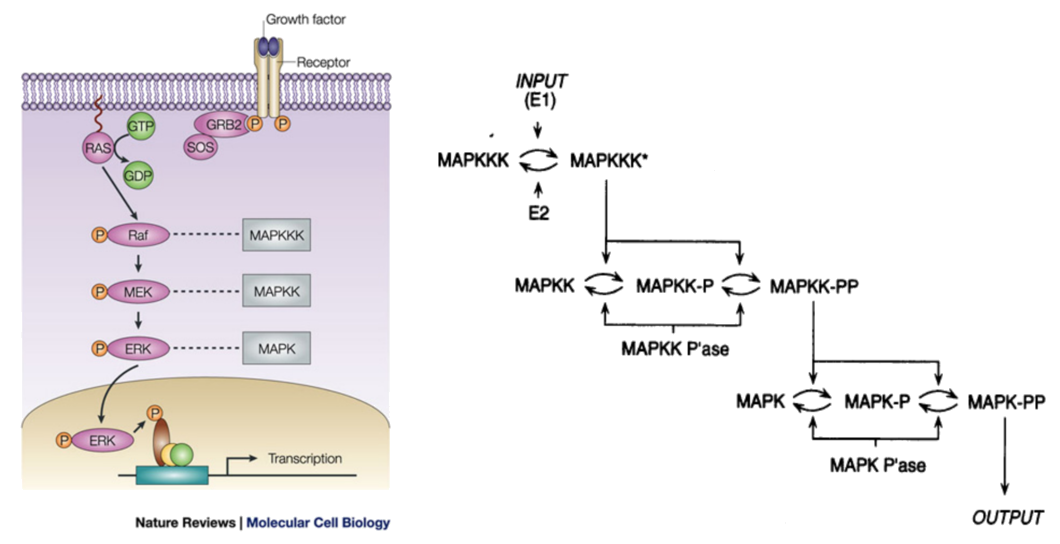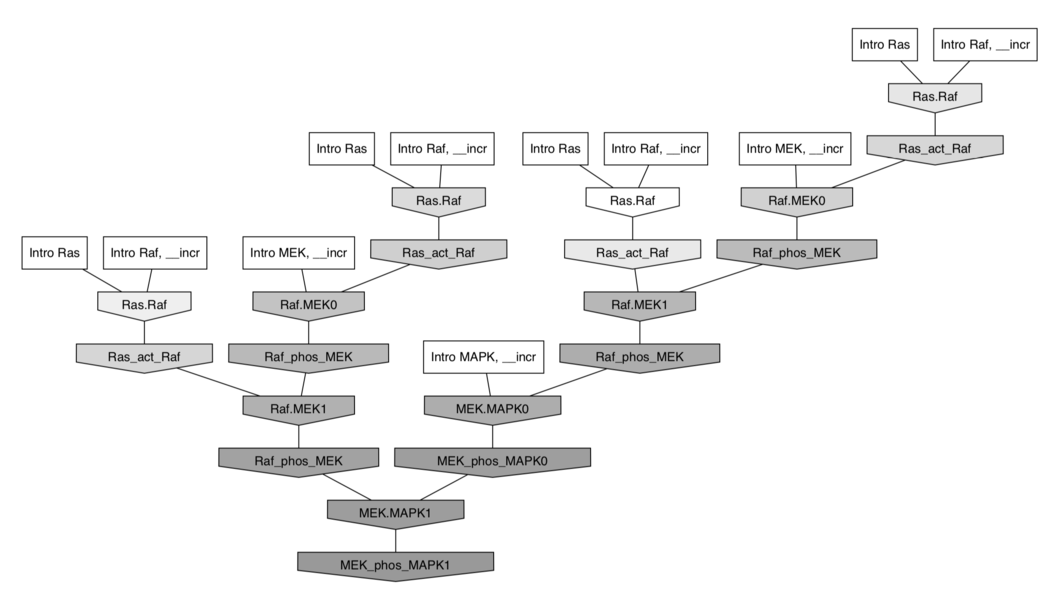# Why does evolution favor deep signalling pathways?

## Example: the MAPK/ERK pathway

Animation courtsey of Ribosome Studio Youtube channelMAPK/ERK Signalling Pathway - Simplified overview

### $⇓ \;$ in KappaSimplified MAPK/ERK causal flow - KaFlow-generated story

### Why does evolution favor deep signalling pathways?

Natural selection: Deeper stories $⟹$ Improving biological fitnessBUT counter-intuitive

• energetically

• probabilistically: Indeed: if

\begin{align*} & 𝔼(\max_i \depth(P_i)) \\ & ≤ 𝔼\Big(\ln\Big(\underbrace{\sum\limits_{ i=0 }^n \exp(\depth(P_i))}_{\; ≝ \; α_n}\Big)\Big) \\ & ≤ \ln \underbrace{𝔼(α_n)}_{\rlap{\substack{= \; 𝔼(α_{n-1}) + 𝔼(\exp(\depth(P_n))) \\ = \, (1 + \frac e i) \, 𝔼(α_{n-1})}}}\\ & = \ln \Big(\prod\limits_{ i=1 }^n \Big(\underbrace{1 + \frac e i}_{≤\, \exp (\frac e i)}\Big) \Big) = e H_n \sim \boxed{e \ln n} \end{align*}

Possible explanations:

Greater depth:
$$\, \\ \\ \\ \begin{cases} \text{ enhances stability } \\ \text{ deletions make pathways non-functional more often than additions do } \end{cases}$$

# II. Theoretical model

Does refinement increase depth?

## Genetic algorithm

An heuristic optimisation that loop on two steps:

• MUTATION: map ancesters to $n$ descendent with random mutations
• SELECTION: filter descendent on their fitness (ERK quantity)

Model biais: biological clock assumption, spontaneous discrete generation, etc …

## III. Refinements

$$\MEK(x_{pp}), \MAPK(x_{p}) ⟶_{τ_b} \MEK(x_{pp}^{\color{DeepPink}{1}}), \MAPK(x_{p}^{\color{DeepPink}{1}})\\ \color{DarkCyan}{\MEK(x_{pp}^1), \MAPK(x_{p}^1) ⟶_{τ_u} \MEK(x_{pp}), \MAPK(x_{p})}\\ \MEK(x_{pp}^1), \MAPK(x_{p}^1) ⟶_{τ_p} \MEK(x_{pp}^1), \color{OrangeRed}{\MAPK(x_{pp}^1)}\\$$

• $\color{DarkCyan}{τ_u}$ too big: unbind before phosphorylation (too liquid)
• $\color{DarkCyan}{τ_u}$ too small: never unbind (too sticky)

## Category of site graphs: $\SGph$

Objects $(V, λ, σ, μ)$:
• $V$: set of nodes
• $λ: V ⟶ 𝒜$: name assignment
• $σ: V ⟶ 𝒫(𝒮)$: site assignment
• $μ: \underbrace{\text{ matching}}_{\rlap{\text{irreflexive symmetric binary relation}}}$ over $\sum\limits_{ v ∈ V } σ(v)$

Morphism $f: (V, λ, σ, μ) ⟶ (V', λ', σ', μ')$:
Name/site/edge-preserving and edge reflecting monomorphism $f: V ⟶ V'$

Signature $Σ: 𝒜 ⟶ 𝒮$:
• $x ≤ Σ \quad ⟺ \quad σ_x(V_x) ⊆ Σ(λ_x(V_x))$
• $Σ ≤ x \quad ⟺ \quad Σ(λ_x(V_x)) ⊆ σ_x(V_x)$

### Epimorphisms

Forgetful functor to the category of graphs:
$$U: \SGph ⟶ \Gph$$

Epimorphisms from $x$ to $y$:
$$[x, y]^e \; ≝ \; \big\lbrace h ∈ \underbrace{\Hom[\SGph]{x, y}}_{\text{denoted by } [x,y]} \; \mid \; h \text{ is an epi (i.e. right-cancellable)} \big\rbrace$$

Lemma:

$$h ∈ \overbrace{\Hom[\SGph]{x, y}}^{\text{denoted by } [x,y]} \text{ is an epi } \\ \; ⟺ \; ∀ c_y ⊆ y \text{ connected component}, \, h^{-1}(c_y) ≠ ∅$$

### Epi-mono factorisation

Factorisation:
$f ∈ [s, x]$ is said to be factored by $t$ if $f = γ ϕ$ for $ϕ ∈ [s, t]^e$ and $γ ∈ [t, x]$

Every iso $α ∈ [t, t']$ conjugates the factorisations $ϕ, γ$ and $ϕ', γ'$:

⟹ Equivalence relation:

$$ϕ, γ \; ≃_{tt'} \; ϕ', γ'$$

The group $[t, t]$ acts freely on $[s, t]^e × [t, x]$ (as we have epis and monos), so by Burnside’s lemma:

$$\vert \underbrace{[s, t]^e × [t, x] / [t, t]}_{\text{denoted by } [s, t]^e ×_{[t, t]} [t, x]} \vert = \frac 1 {\vert [t,t] \vert} \sum\limits_{ (ϕ, γ) ∈ [s, t]^e × [t, x]} \underbrace{\vert Stab_{[t, t]}((ϕ, γ)) \vert}_{= 1} \\ = \vert [s, t]^e × [t, x] \vert/\vert [t,t] \vert$$

### Object refinement

A refinement $Σ(s)$ of $s ≤ Σ$ under $Σ$:
is a set comprised of one element per isomorphism class in $\lbrace t : Σ \; \mid \; [s, t]^e ≠ ∅ \rbrace$

Theorem: if $s ≤ Σ$ and $x : Σ$:

$$[s,x] \; ≅ \; \sum\limits_{ t ∈ Σ(s) } [s, t]^e ×_{[t,t]} [t, x]$$

### Rule refinement

Well defined atomic action (in a rewriting rule):

Labelled transition:

$$x \quad ⟶_f^R \quad f(α) \cdot x$$

where

• the rule $R \; ≝ \; \underbrace{s}_{\text{object}}, \underbrace{α}_{\text{action}}, \underbrace{τ}_{\text{rate}}$, whose activity $a(x, r) \; ≝ \; τ \vert [s, x] \vert$
• $f ∈ [s, x]$

If $θ$ is an iso: $θ(r) \; ≝ \; θ(s), θ(α), τ$ satisfies:

$$x \, ⟶_f^R \, f(α) \cdot x \qquad ⟺ \qquad x \, ⟶_{fθ^{-1}}^{θ(R)} \, fθ^{-1}(θ(α)) \cdot x$$

Rule refinement:
If $s ≤ Σ$, the refinement of the rule $R \; ≝ \; s, α, τ$ under $Σ$ is:

$$Σ(s, α, τ) \; ≝ \; (t, ϕ(α), τ)_{t ∈ Σ(s), ϕ ∈ [s,t]^e/[t,t]}$$

# IV. Methods

Our naive code implementation was split between:

• KaSimir: Genetic algorithm implementation, using KaSim to compute fitness
• KaStorama: wrapper around KaStor to retreive causal story depth from KaSim traces
• KaRapuce: Slitghly change rates of refined rules as mutation event

https://github.com/yvan-sraka/KaSimir

Demo time!

# Bibliography

• P. Boutillier, J. Feret, J. Krivine, and W. Fontana, “The Kappa Language
and Kappa Tools,” p. 52.

• “Signaling Pathways,” Tocris Bioscience. https://www.tocris.com/signaling-pathways.

• V. Danos, J. Feret, W. Fontana, R. Harmer, and J. Krivine, “Rule-Based
Modelling, Symmetries, Refinements,” in Formal Methods in Systems
Biology
, vol. 5054, J. Fisher, Ed. Berlin, Heidelberg: Springer Berlin
Heidelberg, 2008, pp. 103–122.

• E. Murphy, V. Danos, J. Féret, J. Krivine, and R. Harmer, “Rule-Based
Modeling and Model Refinement,” in Elements of Computational Systems
Biology
, H. M. Lodhi and S. H. Muggleton, Eds. Hoboken, NJ, USA: John
Wiley & Sons, Inc., 2010, pp. 83–114.

• “List of signalling pathways,” Wikipedia. 30-Nov-2016.